# kaggle_course_Interactive_Maps（3）交互式html

Interactive_Maps 3

import pandas as pd
import geopandas as gpd
import math
import folium
from folium import Choropleth, Circle, Marker
from folium.plugins import HeatMap, MarkerCluster
one tip

We define a function embed_map() for displaying interactive maps. It accepts two arguments: the variable containing the map, and the name of the HTML file where the map will be saved.

This function ensures that the maps are visible in all web browsers.

def embed_map(m, file_name):
from IPython.display import IFrame
m.save(file_name)
return IFrame(file_name, width='100%', height='500px')
1.simplest 的 map
# Create a map
m_1 = folium.Map(location=[42.32,-71.0589], tiles='openstreetmap', zoom_start=10)
# Display the map
m_1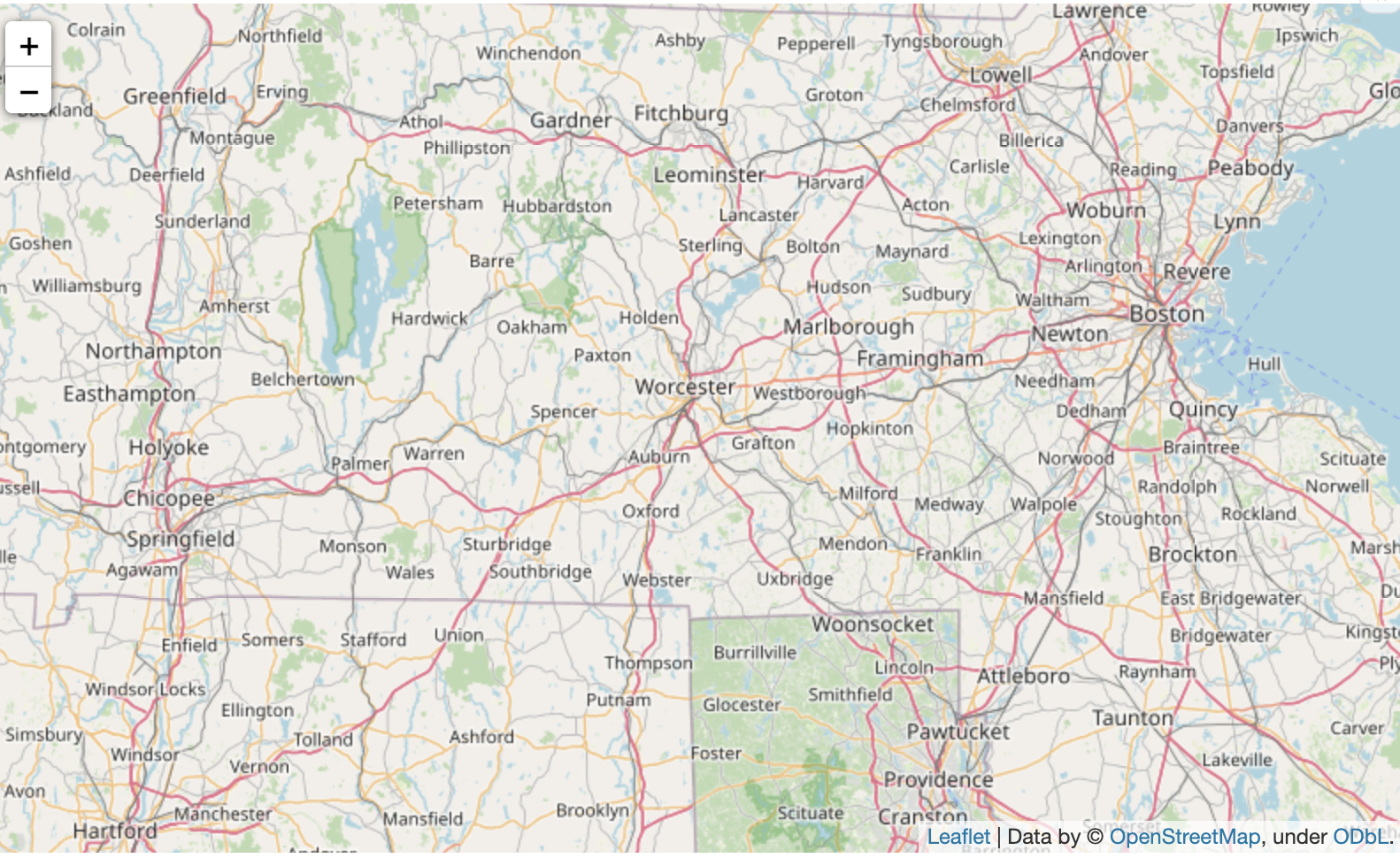Several arguments customize the appearance of the map:

location sets the initial center of the map. We use the latitude (42.32° N) and longitude (-71.0589° E) of the city of Boston.tiles changes the styling of the map; in this case, we choose the OpenStreetMap style. If you’re curious, you can find the other options listed here.zoom_start sets the initial level of zoom of the map, where higher values zoom in closer to the map.

Take the time now to explore by zooming in and out, or by dragging the map in different directions.

2.map+点

folium.Marker We add markers to the map with folium.Marker(). Each marker below corresponds to a different robbery.

# Create a map
m_2 = folium.Map(location = [42.32, -71.0589] , tiles = 'cartodbpositron' , zoom_start = 13)
# Add points to the map
for idx , row in daytime_robberies.iterrows():
Marker([row['Lat'] , row['Long']]).add_to(m_2)
m_2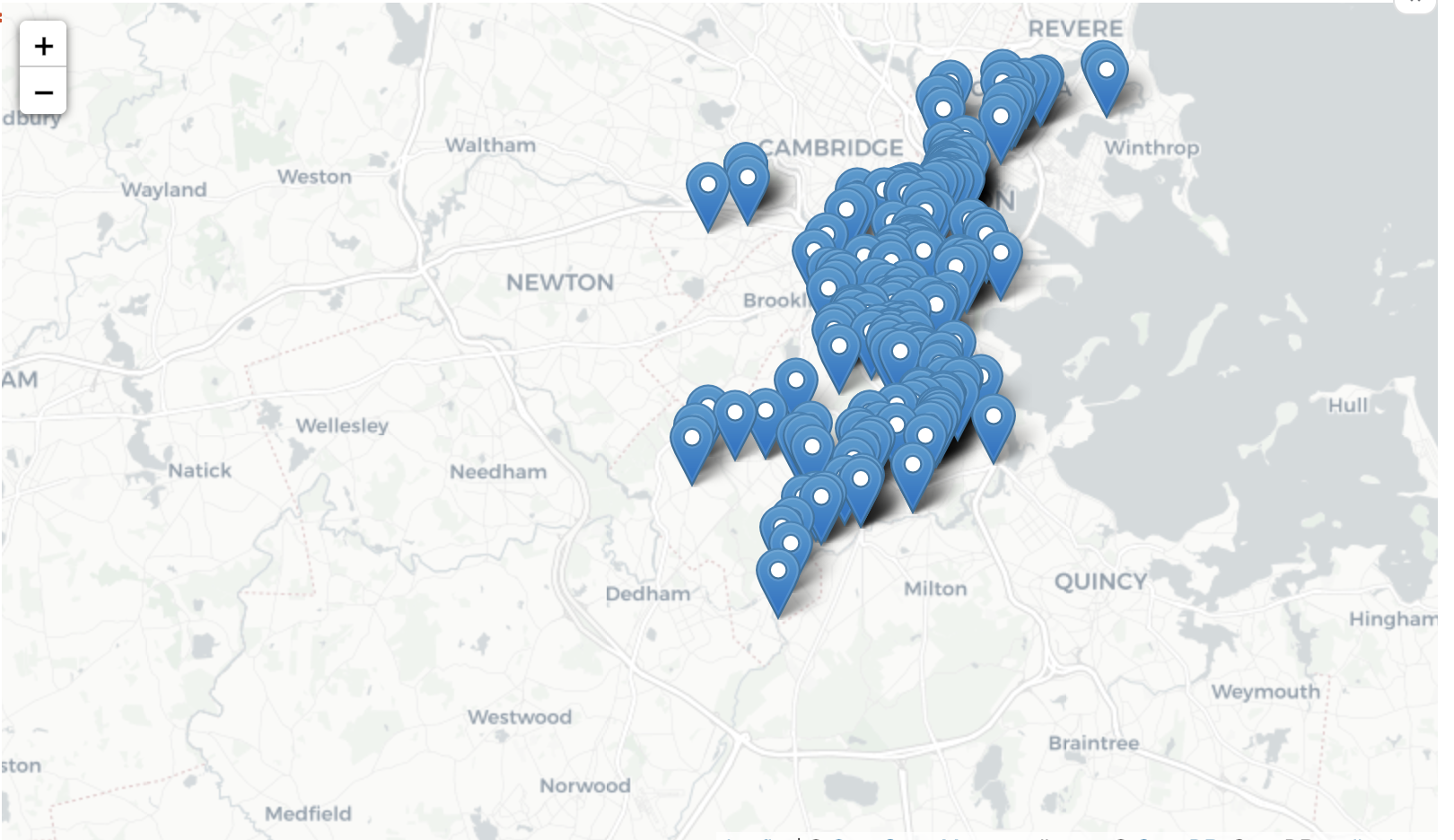3.map+聚集起来的点

folium.plugins.MarkerCluster If we have a lot of markers to add, folium.plugins.MarkerCluster() can help to declutter the map. Each marker is added to a MarkerCluster object.

# Create the map
m_3 = folium.Map(location=[42.32,-71.0589], tiles='cartodbpositron', zoom_start=13)
# Add points to the map
mc = MarkerCluster()
for idx, row in daytime_robberies.iterrows():
if not math.isnan(row['Long']) and not math.isnan(row['Lat']):
mc.add_child(Marker([row['Lat'], row['Long']]))
m_3.add_child(mc)
# Display the map
m_3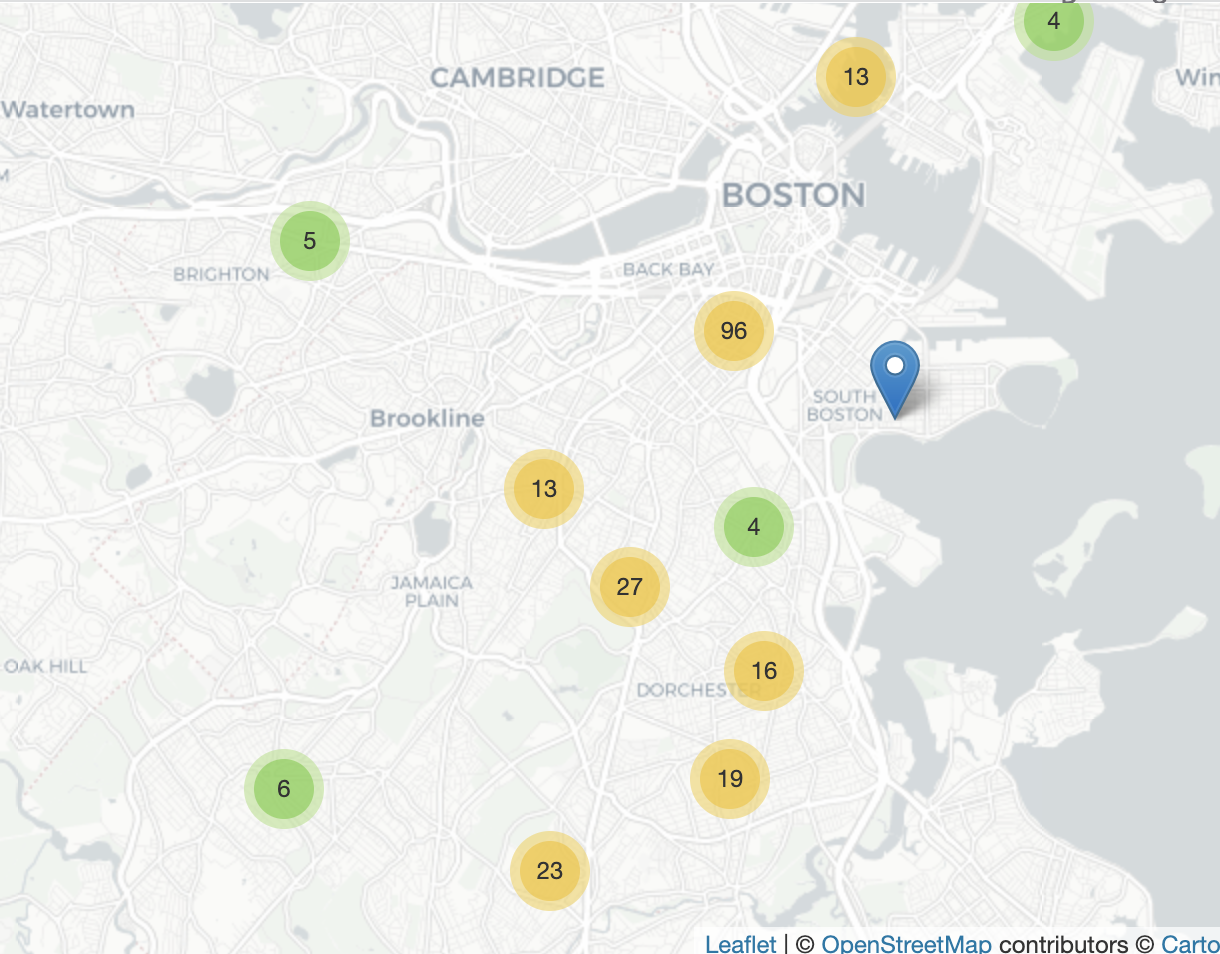4.map+根据数量多少变化颜色的点

Bubble maps

A bubble map uses circles instead of markers. By varying the size and color of each circle, we can also show the relationship between location and two other variables.

We create a bubble map by using folium.Circle() to iteratively add circles. In the code cell below, robberies that occurred in hours 9-12 are plotted in green, whereas robberies from hours 13-17 are plotted in red.

# Create a base map
m_4 = folium.Map(location=[42.32,-71.0589], tiles='cartodbpositron', zoom_start=13)
def color_producer(val):
if val <= 12:
return 'forestgreen'
else:
return 'darkred'
# Add a bubble map to the base map
for i in range(0,len(daytime_robberies)):
Circle(
location=[daytime_robberies.iloc[i]['Lat'], daytime_robberies.iloc[i]['Long']],
radius=20,
color=color_producer(daytime_robberies.iloc[i]['HOUR'])).add_to(m_4)
# Display the map
m_4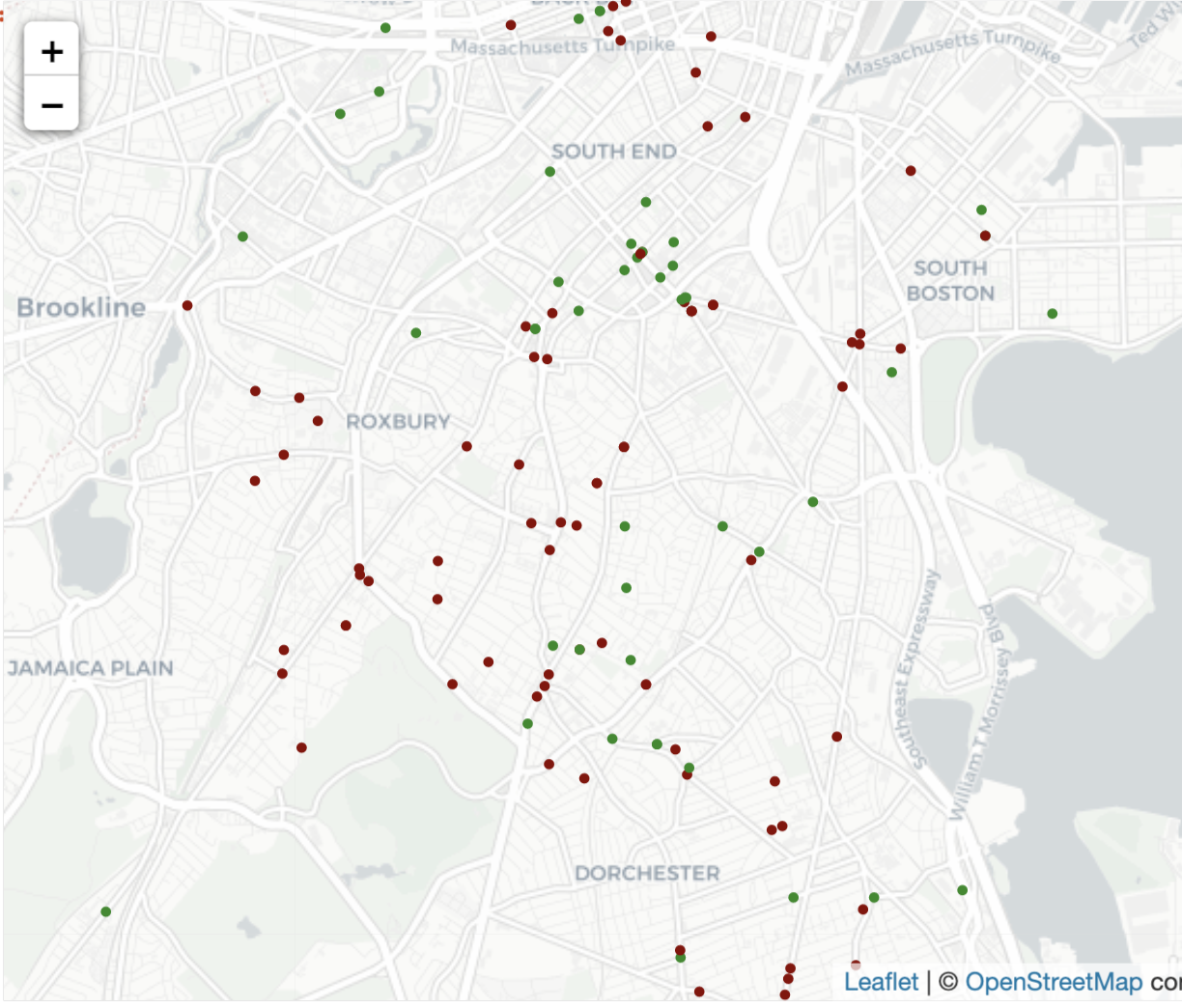Note that folium.Circle() takes several arguments:

location is a list containing the center of the circle, in latitude and longitude.radius sets the radius of the circle.Note that in a traditional bubble map, the radius of each circle is allowed to vary. We can implement this by defining a function similar to the color_producer() function that is used to vary the color of each circle.color sets the color of each circle.The color_producer() function is used to visualize the effect of the hour on robbery location.
5.map+热力图
# Create a base map
m_5 = folium.Map(location=[42.32,-71.0589], tiles='cartodbpositron', zoom_start=12)
# Add a heatmap to the base map
HeatMap(data=crimes[['Lat', 'Long']], radius=10).add_to(m_5)
# Display the map
m_5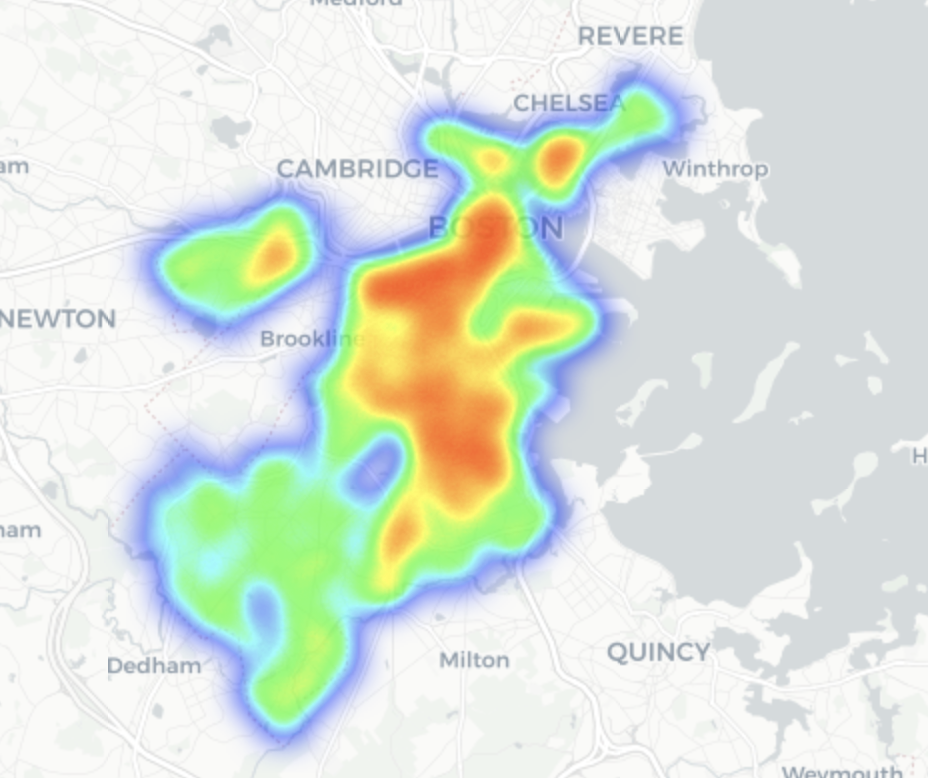As you can see in the code cell above, folium.plugins.HeatMap() takes a couple of arguments:

data is a DataFrame containing the locations that we’d like to plot.radius controls the smoothness of the heatmap. Higher values make the heatmap look smoother (i.e., with fewer gaps).
6.map+区域密度显示图

Choropleth maps

To understand how crime varies by police district, we’ll create a choropleth map.

# Create a base map
m_6 = folium.Map(location=[42.32,-71.0589], tiles='cartodbpositron', zoom_start=12)
# Add a choropleth map to the base map
Choropleth(geo_data=districts.__geo_interface__,
data=plot_dict,
key_on="feature.id",
fill_color='YlGnBu',
legend_name='Major criminal incidents (Jan-Aug 2018)'
).add_to(m_6)
# Display the map
m_6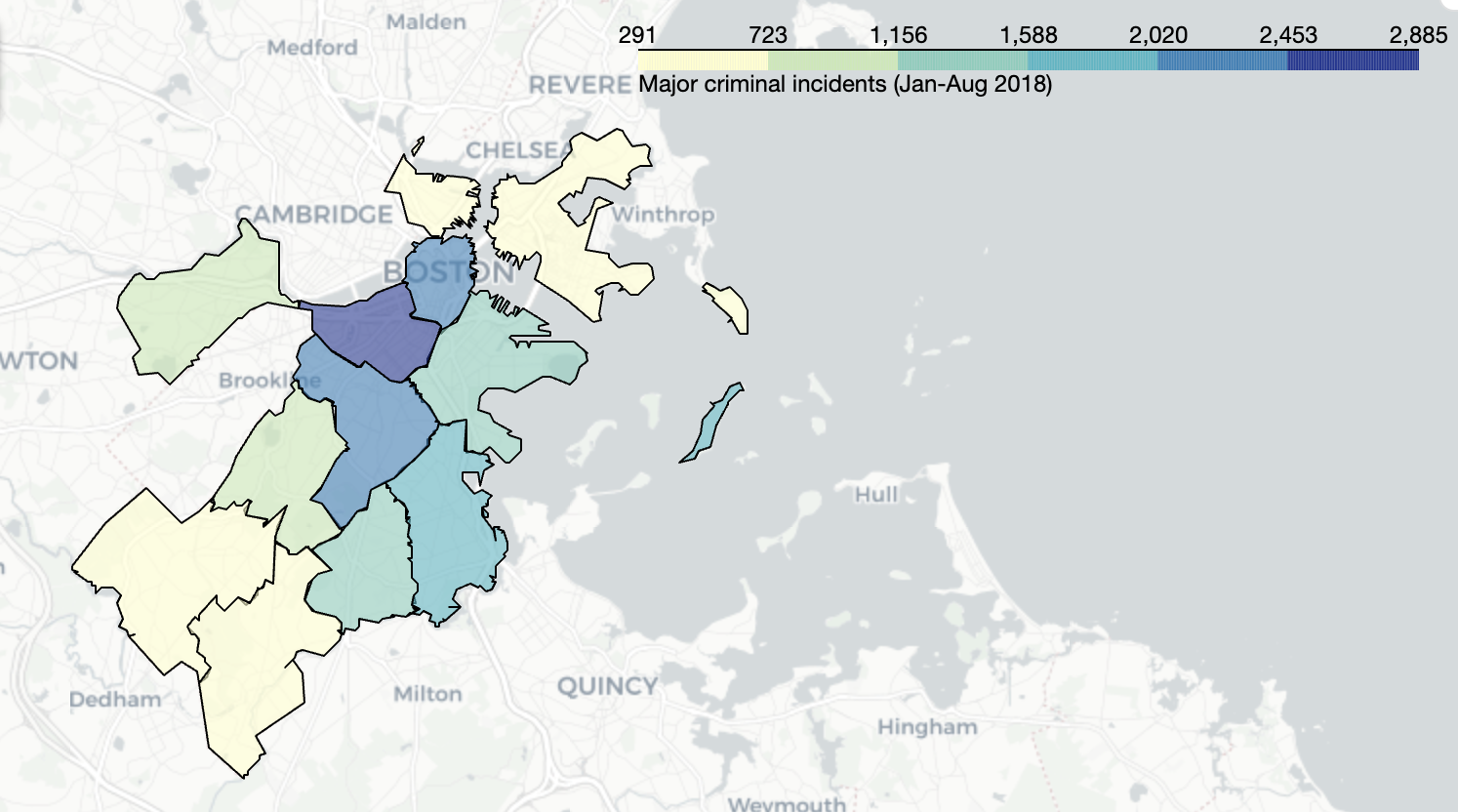Note that folium.Choropleth() takes several arguments:

geo_data is a GeoJSON FeatureCollection containing the boundaries of each geographical area.In the code above, we convert the districts GeoDataFrame to a GeoJSON FeatureCollection with the __geo_interface__ attribute.data is a Pandas Series containing the values that will be used to color-code each geographical area.key_on will always be set to feature.id.This refers to the fact that the GeoDataFrame used for geo_data and the Pandas Series provided in data have the same index. To understand the details, we’d have to look more closely at the structure of a GeoJSON Feature Collection (where the value corresponding to the “features” key is a list, wherein each entry is a dictionary containing an “id” key).fill_color sets the color scale.legend_name labels the legend in the top right corner of the map.
Execrise:
1.地震和板块的边界
# Create a base map with plate boundaries
m_1 = folium.Map(location=[35,136], tiles='cartodbpositron', zoom_start=5)
for i in range(len(plate_boundaries)):
folium.PolyLine(locations=plate_boundaries.coordinates.iloc[i], weight=2, color='black').add_to(m_1)
# Your code here: Add a heatmap to the map
HeatMap(data = earthquakes[['Latitude' , 'Longitude']] , radius = 15).add_to(m_1)
# Uncomment to see a hint
q_1.a.hint()
# Show the map
embed_map(m_1, 'q_1.html')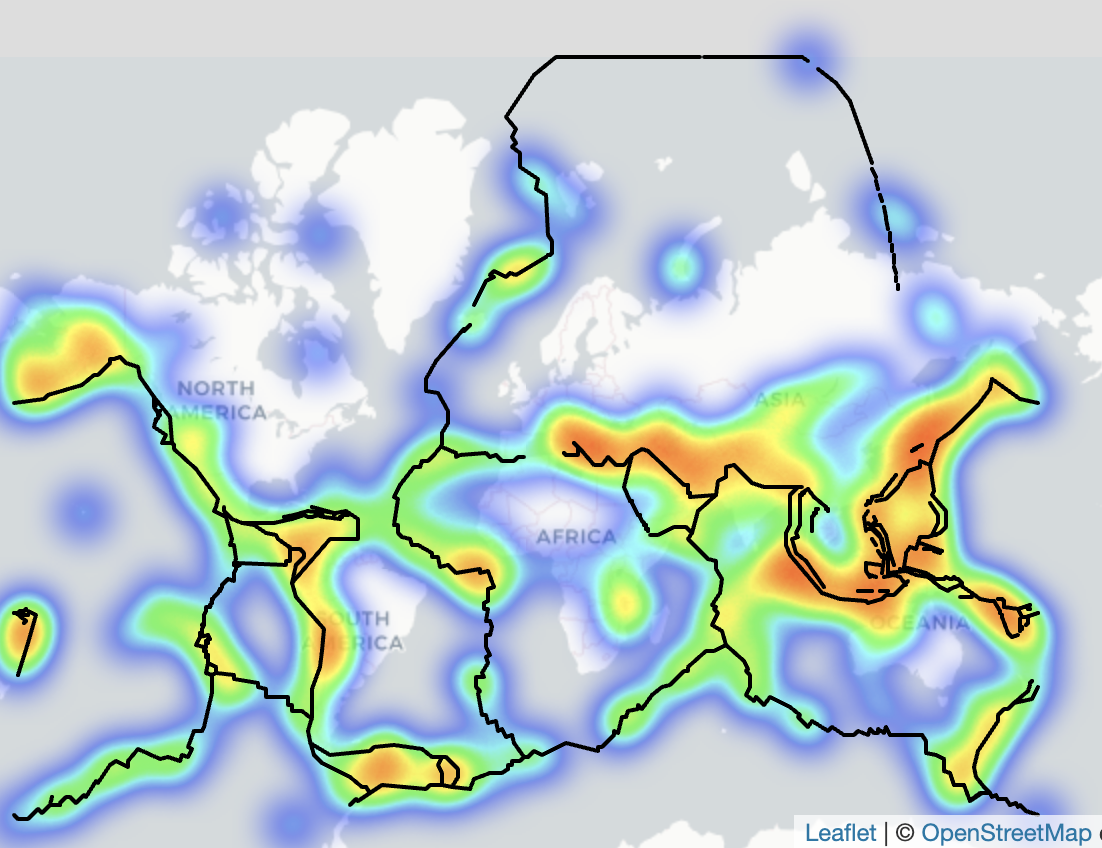2.日本人口密度分布
# GeoDataFrame with prefecture boundaries
prefectures = gpd.read_file("../input/geospatial-learn-course-data/japan-prefecture-boundaries/japan-prefecture-boundaries/japan-prefecture-boundaries.shp")
prefectures.set_index('prefecture', inplace=True)
prefectures.head()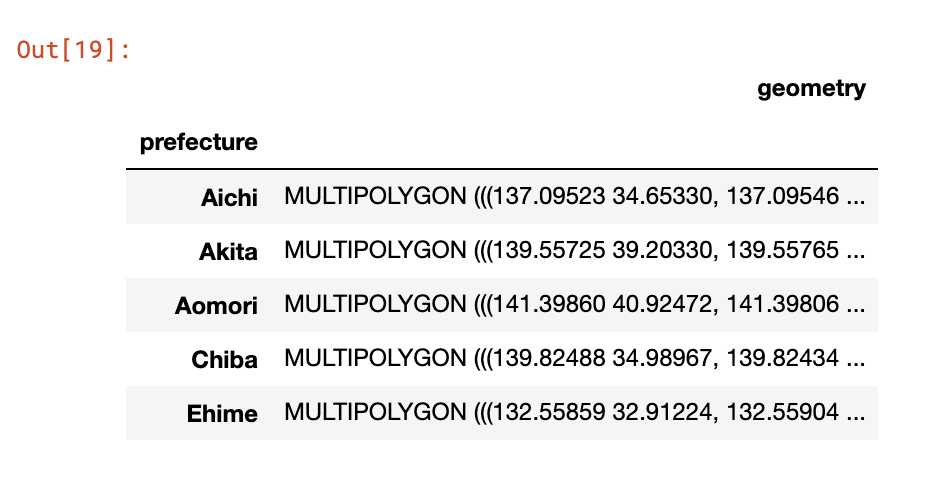# DataFrame containing population of each prefecture
population = pd.read_csv("../input/geospatial-learn-course-data/japan-prefecture-population.csv")
population.set_index('prefecture', inplace=True)
# Calculate area (in square kilometers) of each prefecture
area_sqkm = pd.Series(prefectures.geometry.to_crs(epsg=32654).area / 10**6, name='area_sqkm')
stats = population.join(area_sqkm)
# Add density (per square kilometer) of each prefecture
stats['density'] = stats["population"] / stats["area_sqkm"]
stats.head()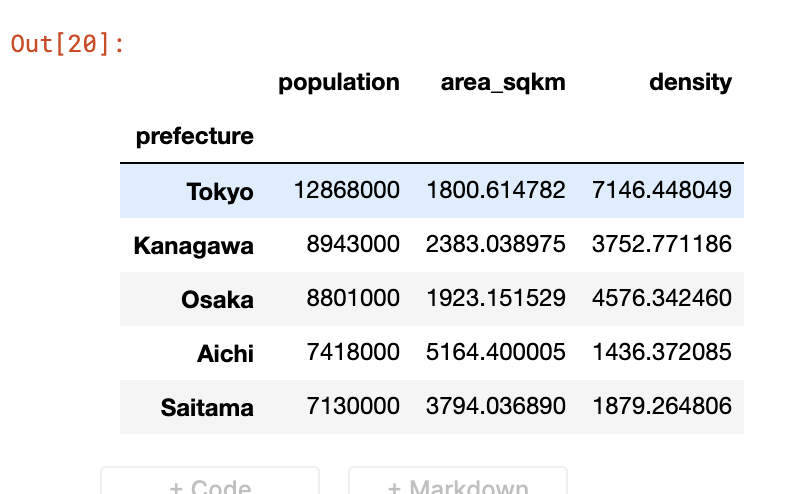# Create a base map
m_3 = folium.Map(location=[35,136], tiles='cartodbpositron', zoom_start=5)
# Your code here: create a choropleth map to visualize population density
# Add a choropleth map to the base map
Choropleth(geo_data=prefectures.__geo_interface__,
data=stats['density'],
key_on="feature.id",
fill_color='YlGnBu',
legend_name='Population density (per square kilometer)'
).add_to(m_3)
# View the map
embed_map(m_3, 'q_3.html')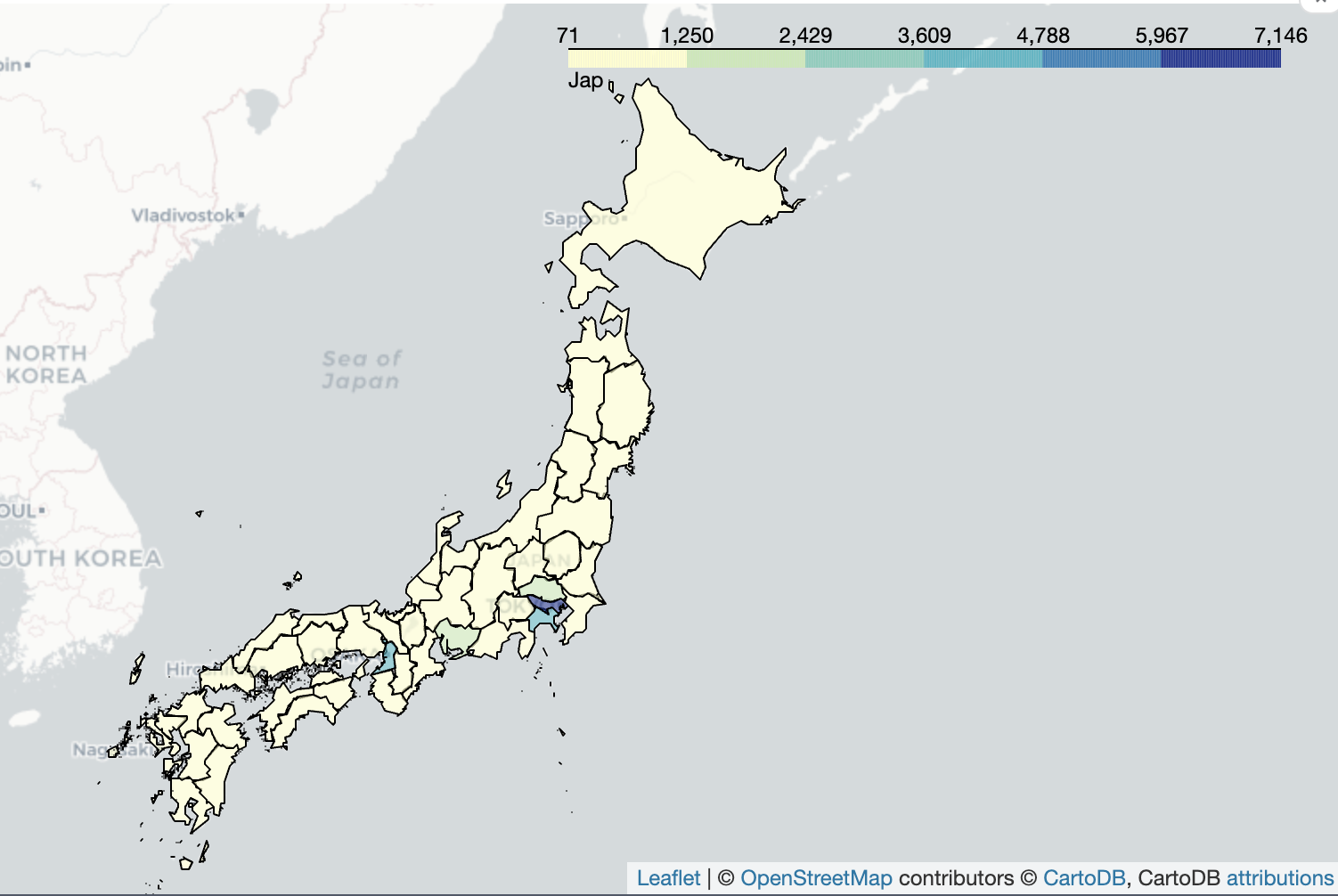3.人口密度和地震强度

Create a map to suggest one prefecture that might benefit from earthquake reinforcement. Your map should visualize both density and earthquake magnitude.

m_4 = folium.Map(location=[35,136], tiles='cartodbpositron', zoom_start=5)
#Create a map
def color_producer(magnitude):
if magnitude > 6.5:
return 'red'
else:
return 'green'
# 画出人口的密度分布图
Choropleth(
geo_data=prefectures['geometry'].__geo_interface__,
data=stats['density'],
key_on="feature.id",
fill_color='BuPu',
legend_name='Population density (per square kilometer)').add_to(m_4)
# 画圆（根据级别来确定 圆的大小的）
for i in range(0,len(earthquakes)):
folium.Circle(
location=[earthquakes.iloc[i]['Latitude'], earthquakes.iloc[i]['Longitude']],
popup=("{} ({})").format(
earthquakes.iloc[i]['Magnitude'],
earthquakes.iloc[i]['DateTime'].year),
radius=earthquakes.iloc[i]['Magnitude']**5.5,
color=color_producer(earthquakes.iloc[i]['Magnitude'])).add_to(m_4)
embed_map(m_4, 'q_4.html')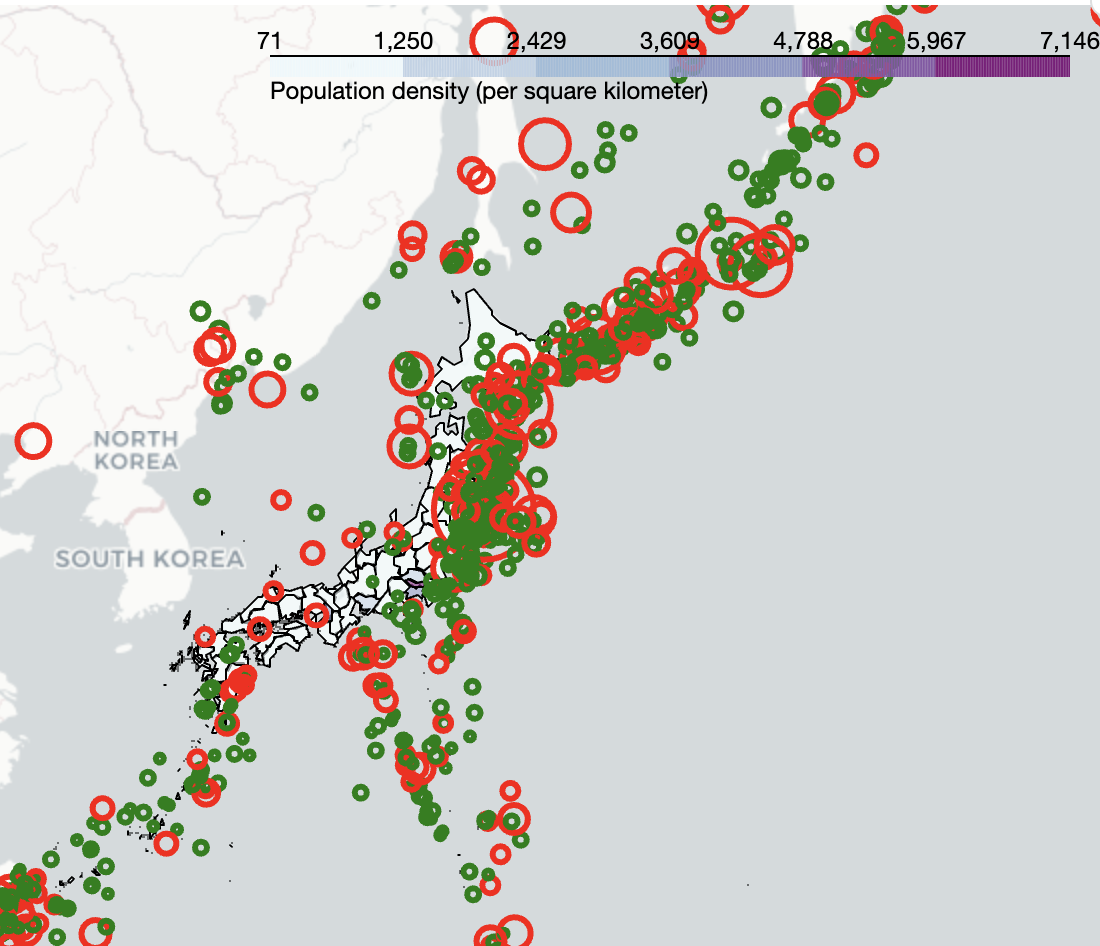## 拍卖算法：Astar算法：Dijkstra；BFS；DFS

BFS：优先搜索深度小的节点，呈现波状的搜索方式，找到的第一条路经就是在最短路径。实现简单可以找到最优解耗时遍历的点多DFS:优先搜索深度大的节点࿰...